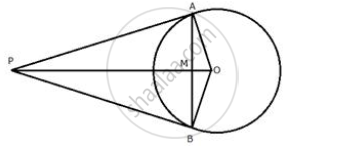Share

From a Point P Outside a Circle, with Centre O, Tangents Pa and Pb Are Drawn. Prove That: (I) ∠Aop = ∠Bop (Ii) Op is the ⊥ Bisector of Chord Ab - ICSE Class 10 - Mathematics

ConceptTangent Properties - If a Line Touches a Circle and from the Point of Contact, a Chord is Drawn, the Angles Between the Tangent and the Chord Are Respectively Equal to the Angles in the Corresponding Alternate Segments

Question

From a point P outside a circle, with centre O, tangents PA and PB are drawn. Prove that:

(i) ∠AOP = ∠BOP
(ii) OP is the ⊥ bisector of chord AB

Solutioni) In Δ AOP and Δ BOP
AP = BP (Tangents from P to the circle)
OP = OP (Common)
OA = OB (Radii of the same circle)
∴ By Side – Side – Side criterion of congruence,
Δ  AOP ≅ Δ BOP
The corresponding parts of the congruent triangle are congruent
⇒ ∠AOP = ∠BOP [by c.p.c.t]

ii) In Δ OAM and  ΔOBM
OA = OB (Radii of the same circle)
∠AOM =∠BOM (Proved ∠AOP  = ∠ BOP )
OM = OM (Common)
∴ By side – Angle – side criterion of congruence,
Δ OAM ≅  ∠OBM
The corresponding parts of the congruent triangles are congruent.
⇒AM = MB
And ∠OMA=∠OMB
But,
∠OMA +∠OMB =180°
∴ ∠OMA = ∠ OMB = 90°
Hence, OM or OP is the perpendicular bisector of chord AB.

Is there an error in this question or solution?

Video TutorialsVIEW ALL 

Solution From a Point P Outside a Circle, with Centre O, Tangents Pa and Pb Are Drawn. Prove That: (I) ∠Aop = ∠`Bop (Ii) Op is the ⊥ Bisector of Chord Ab Concept: Tangent Properties - If a Line Touches a Circle and from the Point of Contact, a Chord is Drawn, the Angles Between the Tangent and the Chord Are Respectively Equal to the Angles in the Corresponding Alternate Segments.
S